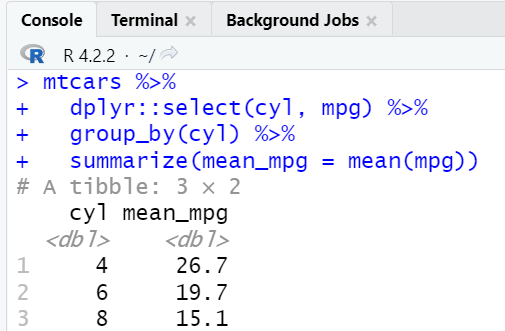# How to Fix: Error in select unused arguments in R

In this tutorial, we will cover how to fix the error in selecting unused arguments in R. Error looks like below in R.

`Error in select(., Species, Sepal.Length) : unused arguments (Species, Sepal.Length)`

This error occurs when we try to use the `select()` function in R's dplyr package, but we also have the some other R package which also has `select()` function. For example MASS package also has select() function. Since R has already loaded `select()` function from the MASS package which causes an error to appear.

## How to reproduce the error

To recreate the error, follow the example below :

```library(dplyr)
library(MASS)

iris %>%
select(Species, Sepal.Length) %>%
group_by(Species) %>%
summarize(mean_sepalLength = mean(Sepal.Length))

Error in select(., Species, Sepal.Length) : unused arguments (Species, Sepal.Length)
```

## How to fix the error

We can fix this error in multiple ways. See the solutions below.

### Solution 1 : Double Colon

By using this code `dplyr::select( )` we have made sure `select( )` function gets loaded from dplyr package instead of MASS package.

```iris %>%
dplyr::select(Species, Sepal.Length) %>%
group_by(Species) %>%
summarize(mean_sepalLength = mean(Sepal.Length))
```
Output
```# A tibble: 3 × 2
Species    mean_sepalLength
1 setosa                 5.01
2 versicolor             5.94
3 virginica              6.59
```
In the code above we used iris dataframe and calculated the average value of `Sepal.Length` variable by different types of `Species`.

### Solution 2 : detach Function

We can also solve this error by unloading the MASS package by using `detach( )` function. This solution is less optimal than the previous one of double colon (::) because we won't be able to use MASS package further in the session if we unload it.

`detach("package:MASS", unload = TRUE)`
Example 2
Let's take one more example to understand it better. Here we are using mtcars dataframe. mpg refers to Miles/(US) gallon and cyl refers to Number of cylinders.
```library(dplyr)
library(MASS)

mtcars %>%
select(cyl, mpg) %>%
group_by(cyl) %>%
summarize(mean_mpg = mean(mpg))

Error in select(., cyl, mpg) : unused arguments (cyl, mpg)
```
Solution

Similar to the previous example, we have used a double colon (::) to ask R to consider the select function from the dplyr package.

```mtcars %>%
dplyr::select(cyl, mpg) %>%
group_by(cyl) %>%
summarize(mean_mpg = mean(mpg))
```
Output
```# A tibble: 3 × 2
cyl mean_mpg

1     4     26.7
2     6     19.7
3     8     15.1
```Related Posts
Share